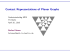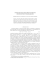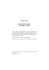# Worksheet 23: Stokes and Divergence Theorems

## Transcription

Worksheet 23: Stokes and Divergence Theorems
```Worksheet 23: Stokes and Divergence Theorems
Recall
• Notation:
n = Φu × Φv
en =
n
||n||
dS = ||n|| dudv
dS = en dS = n dudv
• The parameterization for a surface z = g(x, y) is given by: Φ(x, y) = hx, y, g(x, y)i
A normal for a surface with this parameterization is: n = h−gx , −gy , 1i.
ZZ
ZZ • Surface Integral of a Scalar Function:
f dS =
f Φ(u, v) ||n|| dudv
S
D
ZZ
• Surface Integral of a Vector Function:
ZZ
F · dS =
S
F Φ(u, v) · n dudv
D
ZZ
• The flux of F across S is given by:
F · dS
S
I
• The circulation of F around C is given by:
F · dr
C
• Stokes’ Theorem says the circulation around the boundary is equal to the flux of the curl across the
surface:
I
ZZ
F · dr =
(∇ × F) · dS
C
S
• Divergence Theorem says the flux across a closed surface is equal to the triple integral of the divergence
over the region bounded by the surface:
ZZ
ZZZ
F · dS =
∇ · F dV
S
W
1. Let F(x, y, z) = hxyz, xy, x2 yzi, and let S be the outward oriented surface consisting of the top and four
sides (but not the bottom) of the box with vertices (±1, ±1, ±1). Use Stokes’ Theorem to calculate the
flux of curl F across S.
2. Let F(x, y, z) = hx + y 2 , y + z 2 , z + x2 i, and let C be the boundary of the triangle with vertices
(1, 0, 0), (0, 1, 0), and (0, 0, 1) that has counter-clockwise orientation when viewed from above. Use Stokes’
Theorem to calculate the circulation of F around C.
3. A particle moves along line segments from the origin to the points (1, 0, 0), (1, 2, 1), (0, 2, 1), and back to
the origin under the influence of the force field F(x, y, z) = hz 2 , 2xy, 4y 2 i. Find the work done on the
particle.
4. Let F(x, y, z) = hex sin y, ex cos y, yz 2 i, and let S be the surface of the box bounded by the planes:
x = 0, x = 1, y = 0, y = 1, z = 0, and z = 2. Use the Divergence Theorem to calculate the flux of F
across S.
5. Let F(x, y, z) = hcos z + xy 2 , xe−z , sin y + x2 zi, and let S be the surface of the solid bounded by the
2
2
paraboloid
Z Z z = x + y and the plane z = 4. Use the Divergence Theorem to calculate the surface
integral
F · dS.
S
6. Let F(x, y, z) = h3x, 2y, 0i, and let S be theZ Zsphere of radius 3 centered at the origin. Use the Divergence
F · dS.
Theorem to calculate the surface integral
S
```

### Calculus D Notes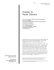### Cultural Divergence and Convergence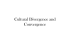### Homework 33: Stokes Theorem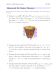### Session 7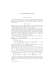### Math 3Z03 Assignment #3 Due: Monday, February 23rd, 2015 Solve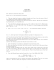### Section 13.2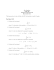### MAT 132 - Calculus II,Practice Problems for Midterm 2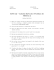### EXPRESS SOLDER SALMIAC### HW8 - UCLA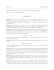### Homework 8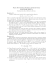### Sample Questions for Final Exam, MATH 2A03/2X03, Spring 2014 Ruipeng Shen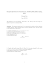### Electromagnetic fields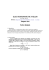### EMT UNIT-1 Q.1 What is the gauss’s law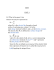### 6 Theme The Integral as an Accumulation Function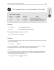### On strongly monotone flows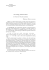### KadisonâSinger conjecture for strongly Rayleigh measures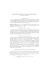### Midterm II Information and Review - Full### A uniform boundedness principle of Pt ak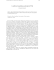### The Manin-Mumford Conjecture for number fields Danny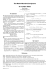### Contact Representations of Planar Graphs